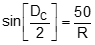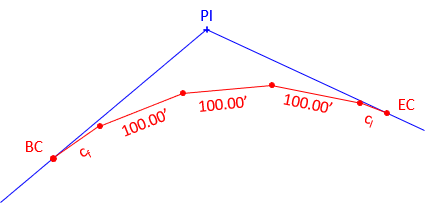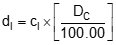### Section 5. Chord Definition

#### a. General

Recall from Section 2 there are two definitions for degree of curvature, Figure C-32.a. Arc Definition b. Chord Definition Figure C-32 Degree of Curvature

Arc definition is the central angle for a 100.00 ft arc; chord is for a 100.00 ft chord.
Since the geometry is slightly different, the mathematical relationship between R and Dc is Equation C-25.Equation C-25

Equations C-3 through C-6 for T, LC, E, and M can be used with no changes.

Equation C-2 for L does not yield the total arc length of the curve from the BC to EC. It returns the sum of the subchords, Figure C-33 and Equation C-26.Figure C-33 Sum of the ChordsEquation C-26

Where:

 cf first partial chord (<100.00 ft) n number of full 100.00 ft chords on the curve cl last partial chord (<100.00 ft)

#### b. Stationing

The same equations are used to compute endpoint stationing for arc and chord definition curves.Equation C-27Equation C-28Equation C-29

A notable difference from the arc definition is that curve stationing is along the chords, not the arcs.

### c. Radial Chord Deflection Method

Because stationing is through the chords, deflection angles to curve points are can be computed by adding incremental deflection angles. The radial chord to each curve point is determined using Equation C-15.

First partial chord, cf

cf is the difference between the first full curve station and the BC station.
The central angle and incremental deflection angles are:Equation C-30Equation C-31

Last partial chord, cl:

cl is the difference between the last full curve station and the EC Back station.
The central angle and incremental deflection angles are:Equation C-32Equation C-33

Nominal chord

The chord between adjacent full stations is 100.00 ft.
The incremental deflection angle is:Equation C-34

Starting with the first incremental deflection angle, the procedure to compute total deflection angle to curve points is:#### d. Example Problem

PI Station is 59+45.00, Δ angle is 30°00'00", a 7°00'00" degree of curvature (chord def) will be used.
Compute the curve table.

(1) Curve components(2) Stationing(3) Incremental deflection angles

first partial chordlast partial chordnominal chord(4) Curve Table
Set up table with stations and incremental deflection angles

 Sta Inc Defl Total Defl Radial Chord EC Bk 61+54.115 1°53'39" 61+00 3°30'00" 60+00 3°30'00" 59+00 3°30'00" 58+00 2°36'21" BC 57+25.544 0°00'00

Compute total deflection angle by adding incremental deflection angles

 Sta Inc Defl Total Defl Radial Chord EC Bk 62+65.715 1°53'39" 15°00'00" 62+00 3°30'00" 13°06'21" 61+00 3°30'00" 9°36'21" 60+00 3°30'00" 6°06'21" 59+00 2°36'21" 2°36'21" BC 58+37.174 0°00'00 0°00'00"
Total deflection angle to EC is 30°00'00"/2 = 15°00'00"  check

Compute radial chord to curve point using:Sta Inc Defl Total Defl Radial Chord EC Bk 62+65.715 1°53'39" 15°00'00" 423.96 62+00 3°30'00" 13°06'21" 371.43 61+00 3°30'00" 9°36'21" 273.34 60+00 3°30'00" 6°06'21" 174.42 59+00 2°36'21" 2°36'21" 74.47 BC 58+37.174 0°00'00 0°00'00" 0.00
Radial chord to EC is 423.96' = LC  check

#### e. Arc Length

Should it be needed, the arc length, LA, from the BC to the EC, can be computed using Equation C-35.Equation C-35

Δr is the Δ angle in radians, Equation C-36.Equation C-36